## CONCEPT

The concept of this project is using vector field to deform moving paths. The end points of one segment is the beginning points of next segment,  which form a recursion.
The parameters which could influence the final result is the position and amount of charge points. the charge value of each points, the density of lines and direction of force vector.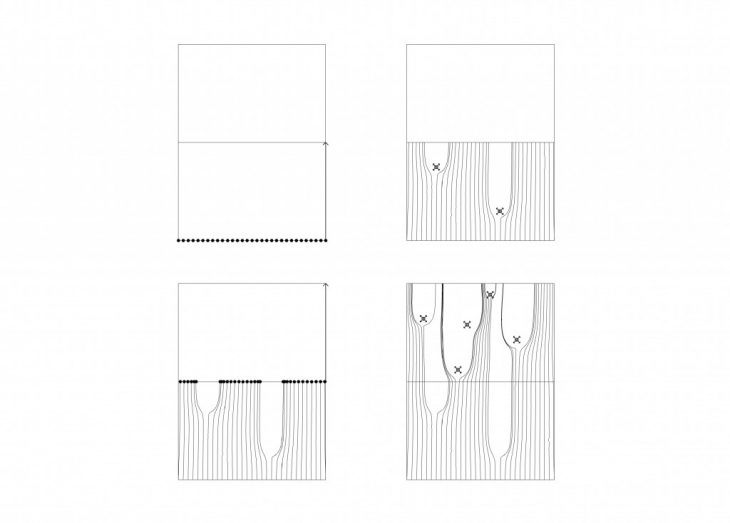## LOCATION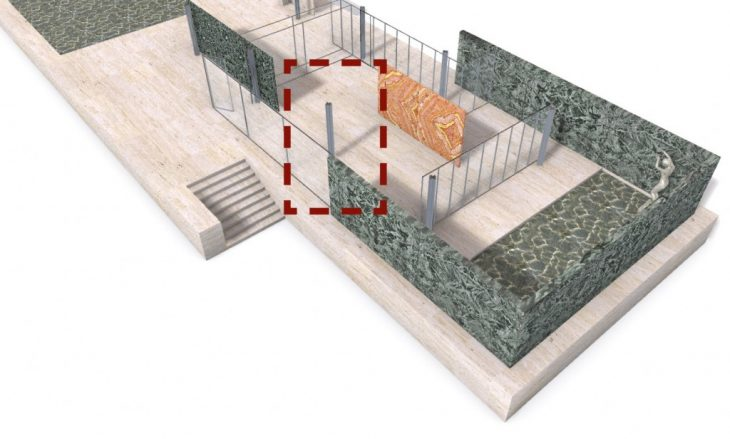## COMPUTATIONAL PROCESS

Base geometry
Use a line perpendicular to the floor as a vector force to create a field.
Create a circle, divide it. Use division points as start points of field lines.
Loop
The element of the loop is lines and end points of lines,
Use interpolate connect start points and move the curve it the center of this segment.
Emit several random points near the interpolated curve, use them as charge points to affect the field line.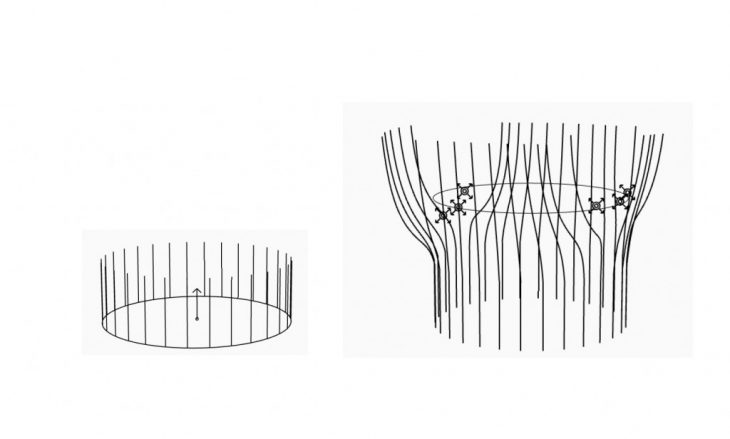## Direction of Vector Force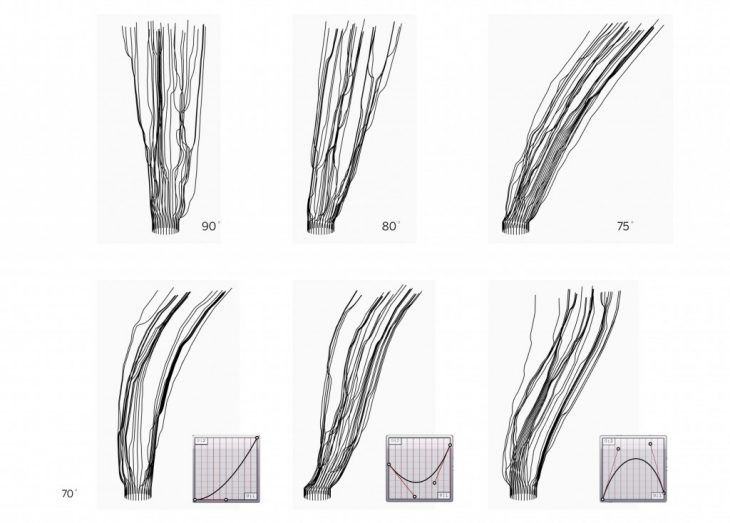## Increase the curve Density to 90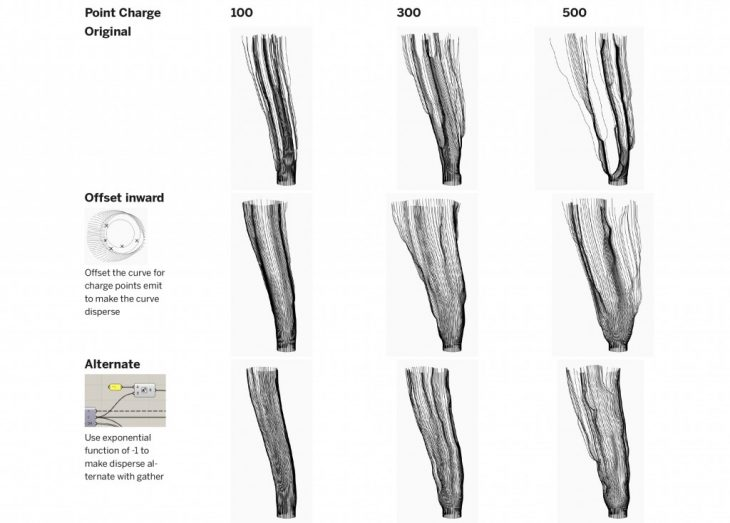Position and value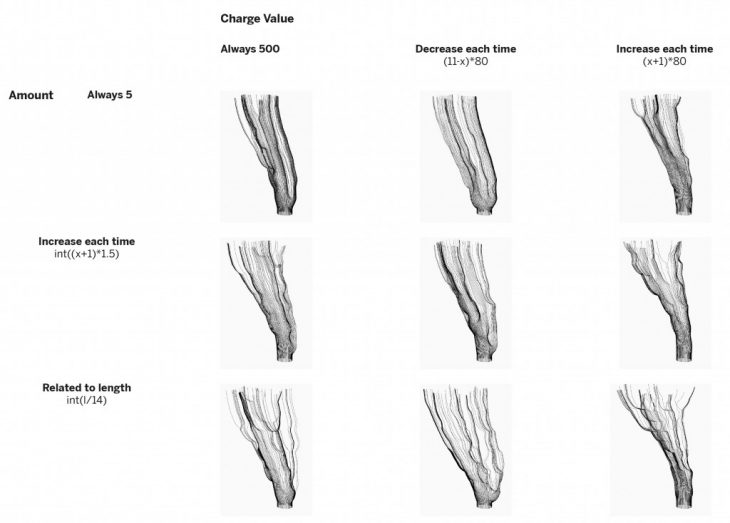Amount and value

## Rebuild Curves## RenderingRecursive Growth is a project of Iaac, Institute for Advanced Architecture of Catalonia developed at Master in Advanced Architecture 2018-2019, during Computational Design course by:
Student: Hongyu Wang
Tutors:   RODRIGO AGUIRRE,
Assistant:  DANIIL KOSHELYUK, Nikoleta mougkasi